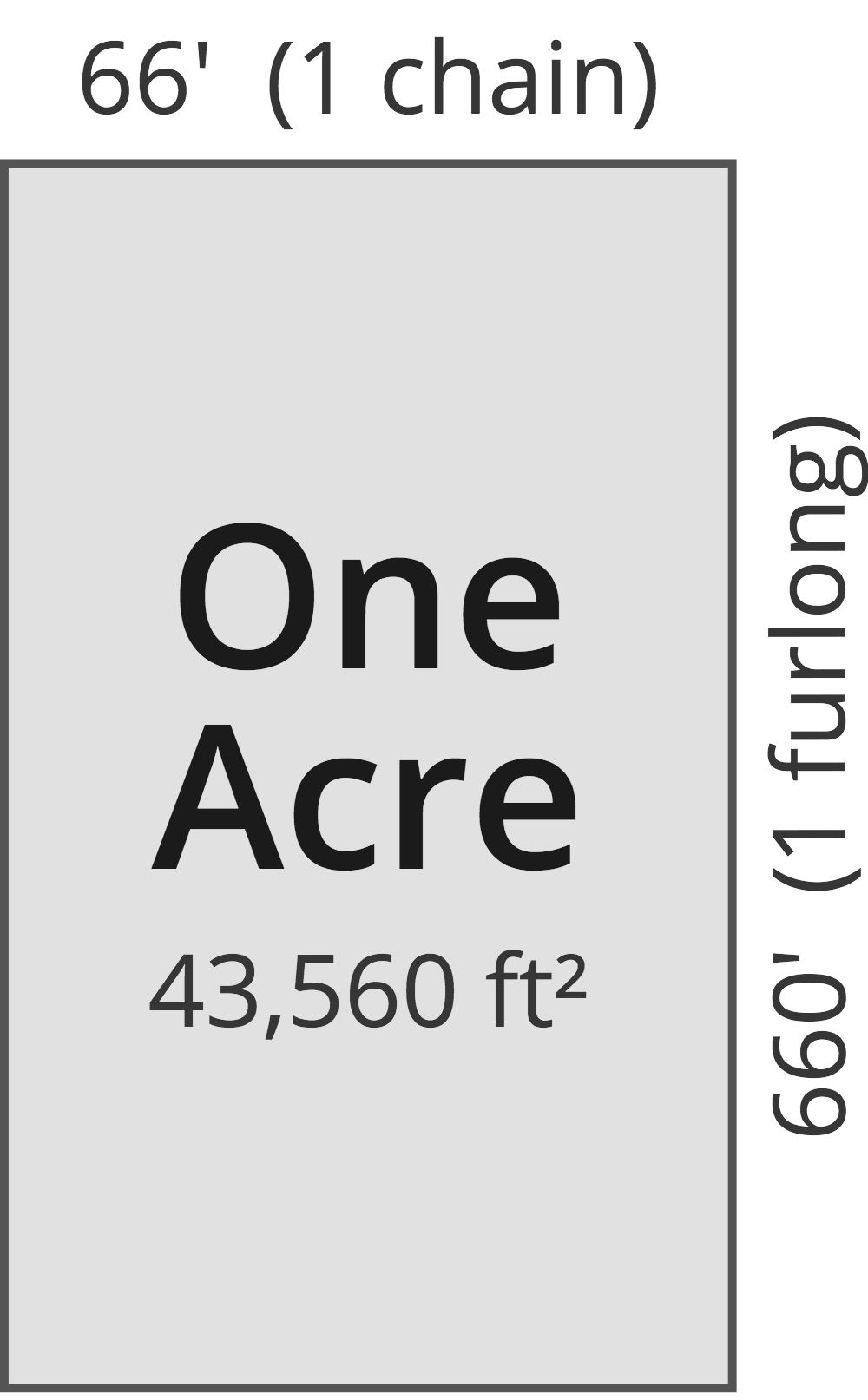# Acres to Square Centimeters Converter

Enter the area in acres below to get the value converted to square centimeters.

(find acres)
Results in Square Centimeters:1 ac = 40,468,564.224 sq cm
1 ac = 4,046.856422 sq m

Do you want to convert square centimeters to acres?

## How to Convert Acres to Square Centimeters

To convert a measurement in acres to a measurement in square centimeters, multiply the area by the following conversion ratio: 40,468,564.224 square centimeters/acre.

Since one acre is equal to 40,468,564.224 square centimeters, you can use this simple formula to convert:

square centimeters = acres × 40,468,564.224

The area in square centimeters is equal to the area in acres multiplied by 40,468,564.224.

For example, here's how to convert 5 acres to square centimeters using the formula above.
square centimeters = (5 ac × 40,468,564.224) = 202,342,821.12 sq cm

### How Many Square Centimeters Are in an Acre?

There are 40,468,564.224 square centimeters in an acre, which is why we use this value in the formula above.

1 ac = 40,468,564.224 sq cm

## What Is an Acre?

One acre is defined as the area equal to a space that is one chain (66 ft) by one furlong (660 ft), or 10 square chains. That's equal to 43,560 square feet or 1/640 of a square mile for those unfamiliar with those units of measure.The acre is a US customary and imperial unit of area. Acres can be abbreviated as ac; for example, 1 acre can be written as 1 ac.

You can use an acreage calculator to measure the area of a plot of land in acres by locating the boundaries on a map.

## What Is a Square Centimeter?

One square centimeter is equivalent to the area of a square with 1 centimeter long sides. One square centimeter is equal to 0.155 square inches or 1/10,000 of a square meter.

The square centimeter, or square centimetre, is a multiple of the square meter, which is the SI derived unit for area. In the metric system, "centi" is the prefix for hundredths, or 10-2. A square centimeter is sometimes also referred to as a square cm. Square centimeters can be abbreviated as sq cm, and are also sometimes abbreviated as cm². For example, 1 square centimeter can be written as 1 sq cm or 1 cm².

## Acre to Square Centimeter Conversion Table

Table showing various acre measurements converted to square centimeters.
Acres Square Centimeters
0.0000001 ac 4.0469 sq cm
0.0000002 ac 8.0937 sq cm
0.0000003 ac 12.14 sq cm
0.0000004 ac 16.19 sq cm
0.0000005 ac 20.23 sq cm
0.0000006 ac 24.28 sq cm
0.0000007 ac 28.33 sq cm
0.0000008 ac 32.37 sq cm
0.0000009 ac 36.42 sq cm
0.00000001 ac 0.404686 sq cm
0.0000001 ac 4.0469 sq cm
0.000001 ac 40.47 sq cm
0.00001 ac 404.69 sq cm
0.0001 ac 4,047 sq cm
0.001 ac 40,469 sq cm
0.01 ac 404,686 sq cm
0.1 ac 4,046,856 sq cm
1 ac 40,468,564 sq cm

## References

1. National Institute of Standards and Technology, Specifications, Tolerances, and Other Technical Requirements for Weighing and Measuring Devices, Handbook 44 - 2019 Edition, https://nvlpubs.nist.gov/nistpubs/hb/2019/NIST.HB.44-2019.pdf